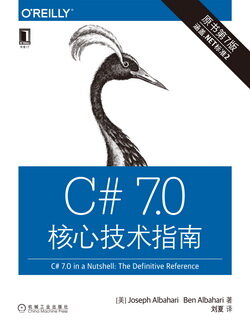# C# 7.0 核心技术指南 (43)：C#语言基础 2.9.2&2.9.3(空表达式)

Console.WriteLine (1)

1 + Console.WriteLine (1)      // Compile-time error

(赋值表达式)

x = x * 5

y = 5 * (x = 2)

a = b = c = d = 0

x *= 2    // equivalent to x = x * 2x <<= 1   // equivalent to x = x << 1

（这条规则的例外是第 4 章中介绍的事件（event）。它的 += 和 -= 运算符会特殊对待并映射至事件的 add 和 remove 访问器上。）